# OO第一单元总结

## 第一次作业总结

### 分析

• 1. 将输入表达式转化为后缀表达式 （展开括号）
• 2. 将后缀表达式计算并化简为顺序结果 （计算结果）

### 基本思路

• 1.递归下降法求后缀表达式

• 首先对表达式进行预处理

1. 去除所有空白字符

2. 第一项为符号，则在表达式开头补 0

3. 在表达式中连续的 +/- 号，整合成一个 +/-

4. 在表达式中 ** 后面紧跟着+ 号，删除之

• 通过利用形式化表述定义，将表达式分成了四个层次：因子（常量或变量）、基本项（幂）、（乘除）、表达式（加减），这四种结构对应了四种对象。我们下面分别进行讨论对这些对象的解析：

• 对于因子（常量或变量）的解析：若下一个字符为单目运算符或者是数字或者是变量 x ，则不断看下一个字符是否是数字。如果不是数字，那么说明下一个符号不属于因子，即对因子的解析结束。

• 对基本项（幂）的解析：基本项由因子的幂次方组成，即为 [加减] 因子 ** 带符号整数 。当解析到一个因子后，若下一个解析到的为 ** ，那么读入这个符号并做处理，然后下一个符号一定是一个带符号整数，否则，说明基本项的解析结束了。

• 对项（乘除）的解析：项由基本项或因子和乘除符号组成，即为 [加减] 因子 | 项 * 因子 ，处理方法与基本项相似。

• 对表达式（加减）的解析：表达式由项和加减符号组成，即为：[加减] 项 | 表达式 加减 项

• 通过题目所给的形式化表述可以表叫容易地建立层次，并且通过方法的互相调用完成解析。递归下降方法的好处在于，它可以通过方法之间的间接递归调用，非常自然地处理嵌套的表达式（即带嵌套括号的表达式）。

• 2.后缀表达式计算

• 1步中所得的后缀表达式中，运算符为 +/-/*/** ，操作数为 带符号整数 / 变量x

• 把所有操作数和运算结果视为一系列单项式之和（多项式）

• 则：

• 对于 带符号整数 ，视为指数为零，系数为带符号整数的值的单项式

• 对于 变量x ，视为指数和系数均为 1 的单项式

• 由于后缀表达式的便利性，因此我们每读到一个运算符，就对最近的两个多项式进行对应运算并返回一个多项式即可

### 具体实现

• #### 读取处理类 Lexer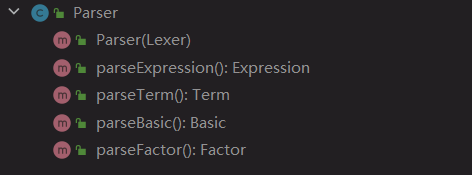• 该类主要目的是对原始表达式进行读取处理，使其按照需要每次使用都能输出一个 操作数/运算符
• 对字符串进行必要的预处理
• 读取数字需要判断正负（即该数前面的 +/- 号（如果有）是否是单目运算符）
• #### 解析类 Parser• 使用递归下降法处理表达式，递归地分别对表达式（Expression）、项（Term）、基本项（Basic）、因子（Factor）进行处理
• 若是识别到左括号 ( 则递归调用 parseExpression() 先处理括号内的内容即可
• #### 多项式类 Item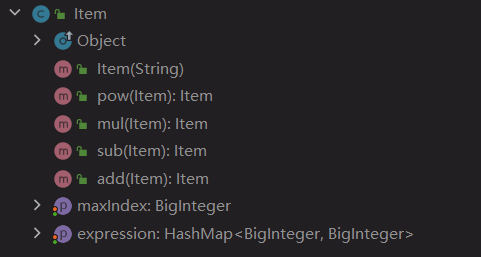• 对于多项式的 +/-/*/** 操作均在该类中使用公开函数实现，需要时根据运算符类型调用即可
• 重写 toString 方法，对保存在该类的各单项式对指数项进行排列后输出
• #### 因子类接口 Factor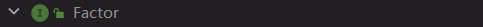• ##### 表达式（加减）类 Expression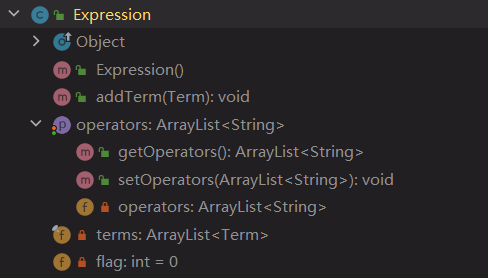• 储存 表达式
• 由于 +- 地位相同，所以让该类自带一个队列（此处用 ArrayList 实现，并用 flag 标记队首
• 重写 toString 方法，把保存在该类中的数逐个输出并用 +/-(队首元素) 相连
• ##### 项（乘除）类 Term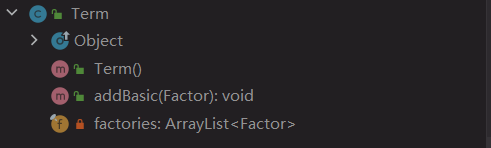• 储存 项
• 重写 toString 方法，把保存在该类中的数逐个输出并用 * 相连
• ##### 基本项（幂）类 Basic• 储存 基本项
• 重写 toString 方法，把保存在该类中的数逐个输出并用 ** 相连
• ##### 常量类 Constant• 储存 常量因子
• ##### 变量类 Variable• 储存 变量因子

### 基于度量的程序结构分析

• 代码规模分析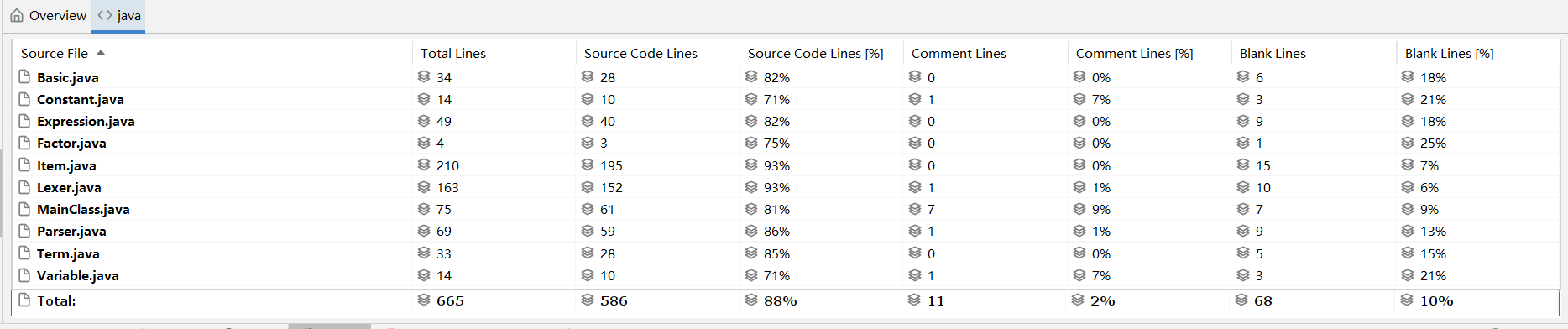• 方法复杂度分析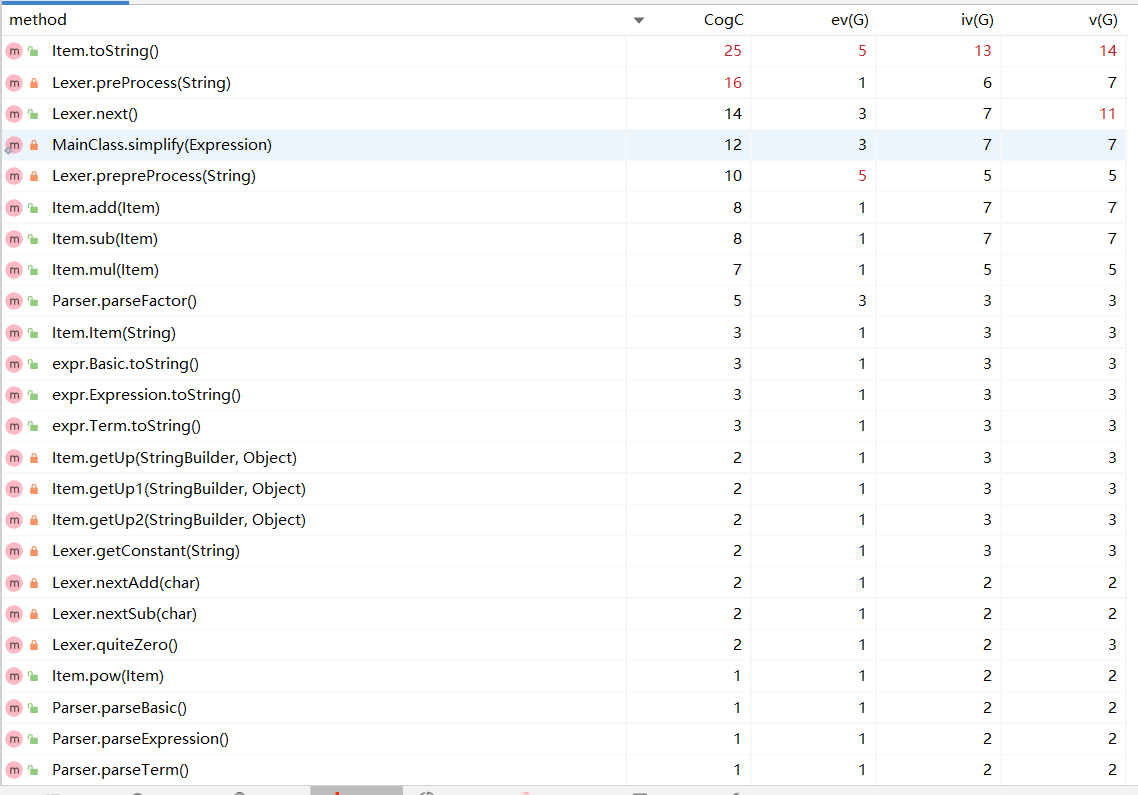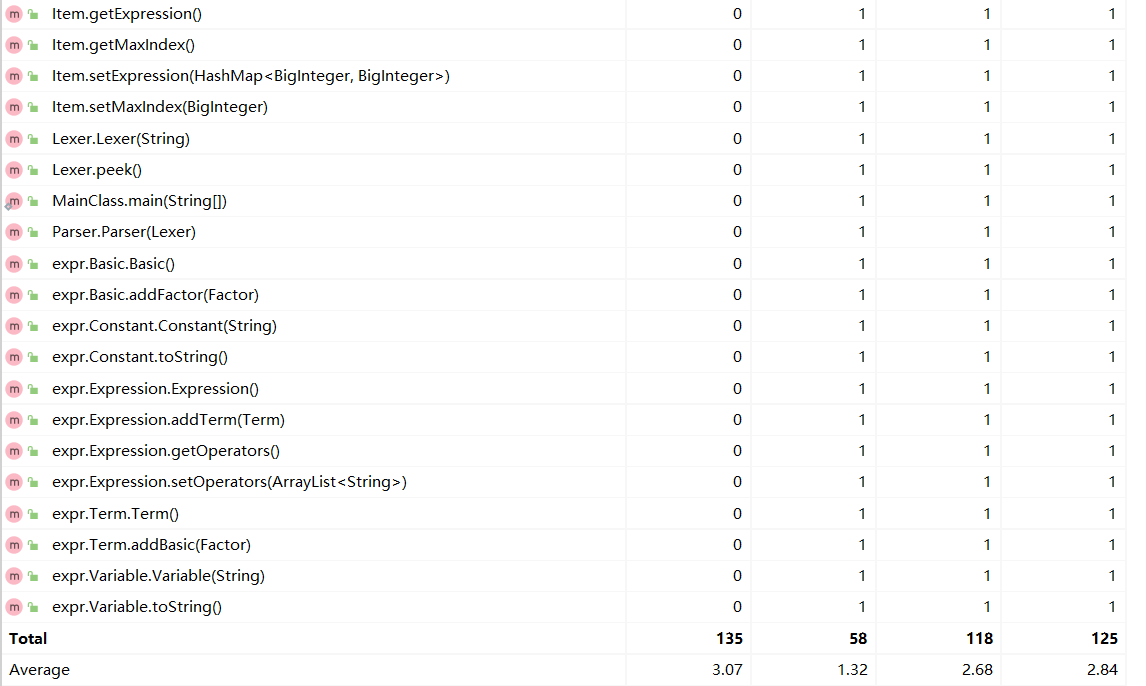• 类复杂度分析1. x**2x*x 替换
2. 把多项式的首个非负项放在表达式首位显示

## 第二次作业总结

### 需求变更

#### 新增内容:

• 三角函数：

• 三角函数 $$\rightarrow$$ 'sin' 空白项 '(' 空白项 因子 空白项 ')' [空白项 指数] | 'cos' 空白项 '(' 空白项 因子 空白项 ')' [空白项 指数]
• 自定义函数

• 自定义函数定义 $$\rightarrow$$ 自定义函数名 '(' 空白项 函数自变量 空白项 [',' 空白项 函数自变量 空白项 [',' 空白项 函数自变量 空白项]] ')' 空白项 '=' 空白项 函数表达式
• 函数自变量 $$\rightarrow$$ 'x' | 'y' | 'z'
• 自定义函数调用 $$\rightarrow$$ 自定义函数名 空白项 '(' 空白项 因子 空白项 [',' 空白项 因子 空白项 [',' 空白项 因子 空白项]] ')'
• 自定义函数名 $$\rightarrow$$ 'f' | 'g' | 'h'
• 求和函数

• 求和函数 $$\rightarrow$$ 'sum' '(' 空白项 i 空白项',' 空白项 常数因子 空白项 ',' 空白项 常数因子 空白项 ',' 空白项 求和表达式 空白项 ')'

#### 数据限制变更:

• 指数变更：

• 指数输入、计算过程中和最终计算结果最高次为 8 $$\rightarrow$$ 指数输入最高次为 8互测对指数无要求 （已于第一次作业开发完成）
• 括号嵌套：

• 本次作业可能会存在多层括号嵌套 （已于第一次作业开发完成）

### 迭代开发

• #### 求和函数类 SumFun• 该类带有一个处理方法，用于字符串的预处理，将输入字符串中所有的 sum 函数展开，返回处理后的字符串
• 如： sum(i, 1, 3, (i*x)) 处理为 ((1*x)+(2*x)+(3*x))
• tips: 直接 replaceAll 的小心 "sin" 里面也有个"i"
• tips: sum 的上下限需要用 BigInter
• #### 自定义函数类 SelfDefineFun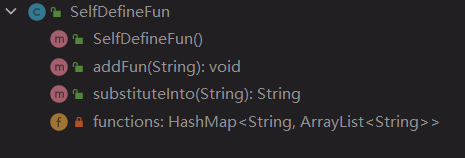• 该类的addFun方法读入一个自定义函数表达式，以 HashMap<String, ArrayList<String>> 的形式保存于该类中
• 如： f(x,y,z)=x+y**2+z**3 存储为 HashMap<f,[x, y, z, (x+y**2+z**3)]>
• 该类亦带有一个处理方法，同样用于字符串的预处理，将本类中所存的所有自定义函数都代入输入字符串中，并返回处理后的字符串
• 如： 自定义函数为：f(x,y,z)=x+y**2+z**3 ; 待处理表达式为：f(sin(x)**2,cos(x),x) ;  处理为 ((sin(x)**2)+(cos(x))**2+(x)**3)
• tips: 需要先替换 x 以免出现 x 的重复代入
• #### 三角函数类 TriFun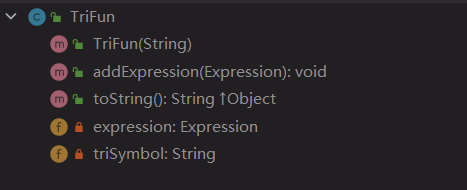• 该类在递归下降算法中位于 Experssion类 之上，具有 addExpression 方法用于递归
• 使用一个字符串用于记录三角函数具体类型（ sin / cos
• 重写 toString 方法，把保存在该类中存储的表达式并输出三角函数类型（视为单目运算符）
• #### 三角函数化简类 TriSimplify• 该类用于将一个 Item 类中符合三角函数化简规则的项进行运算并化简，返回一个处理后的 Item

• #### 读取处理类 Lexer

• 增加 sincos 识别
• 变更识别变量 x 为识别所有自变量、
• #### 解析类 Parser

• 递归下降算法输出后缀表达式时加入三角函数类，输出时将三角函数视为单目运算符（即增加 parseTriFun 方法）
• 变更识别变量 x 为识别所有自变量
• #### 多项式类 Item

• 需要重构： 该类在第一次作业中只涉及变量 x 的计算，在本次作业中要涉及多变量计算（把三角函数作用于表达式后的整体视作一个自变量（原因：无法与 x 合并，具有自己独立的系数和指数） ）
• 将多项式以 HashMap<HashMap<String, BigInteger>, BigInteger> 的形式保存于该类中
• 如：5*x*sin(x**2)**2 保存为 HashMap<HashMap(<x,1> & <sin(x**2),2>),5>
• 根据新类型重构 add/sub/mul/pow (+/-/*/**) 方法
• 新增 sin/cos 方法，作用为把表达式用 sin()/cos() 包裹并定义为新自变量

### 基于度量的程序结构分析

• 代码规模分析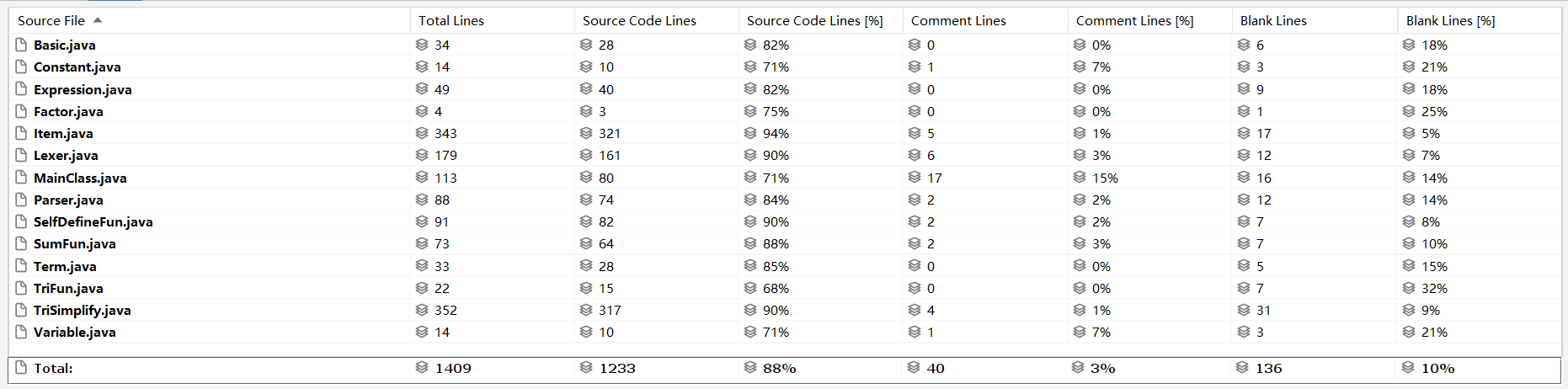• 方法复杂度分析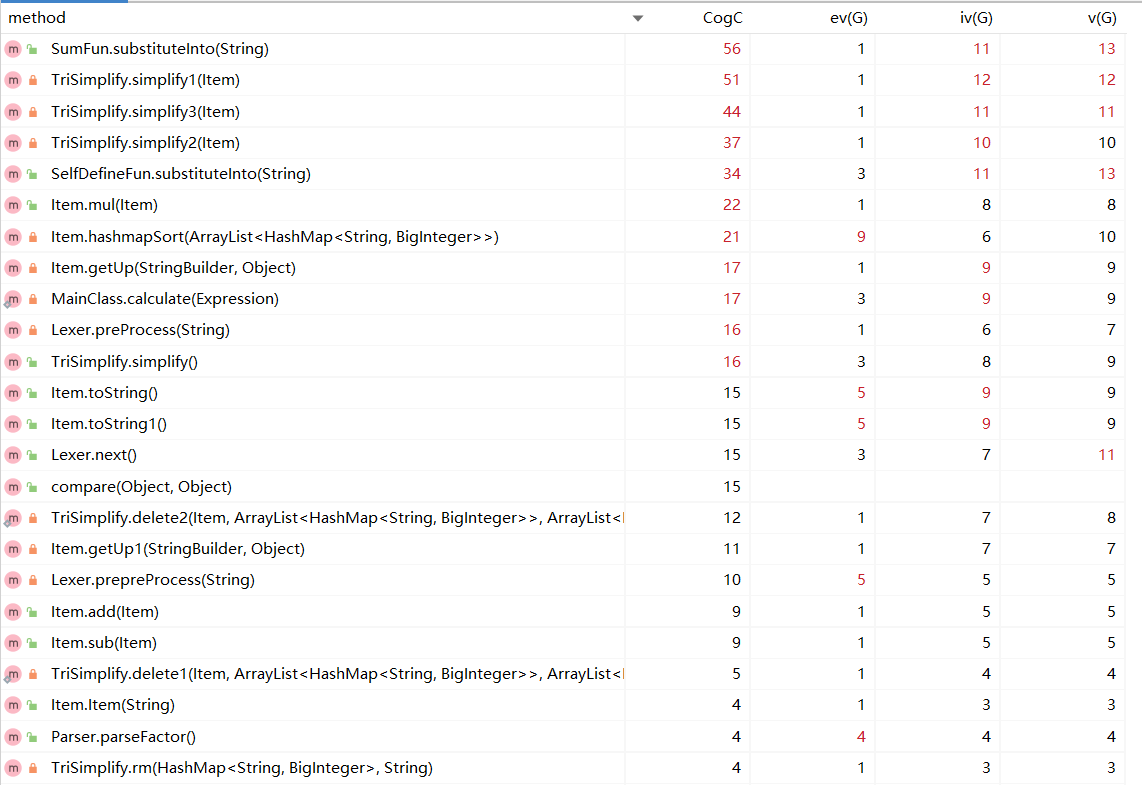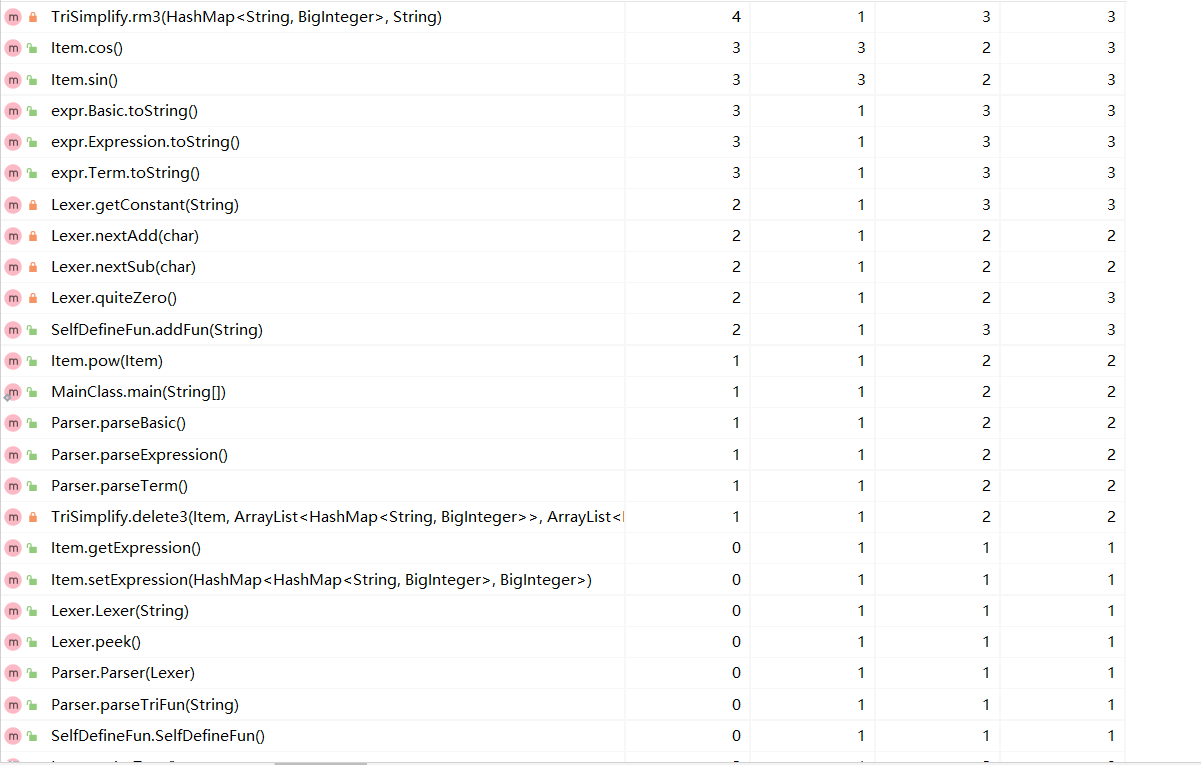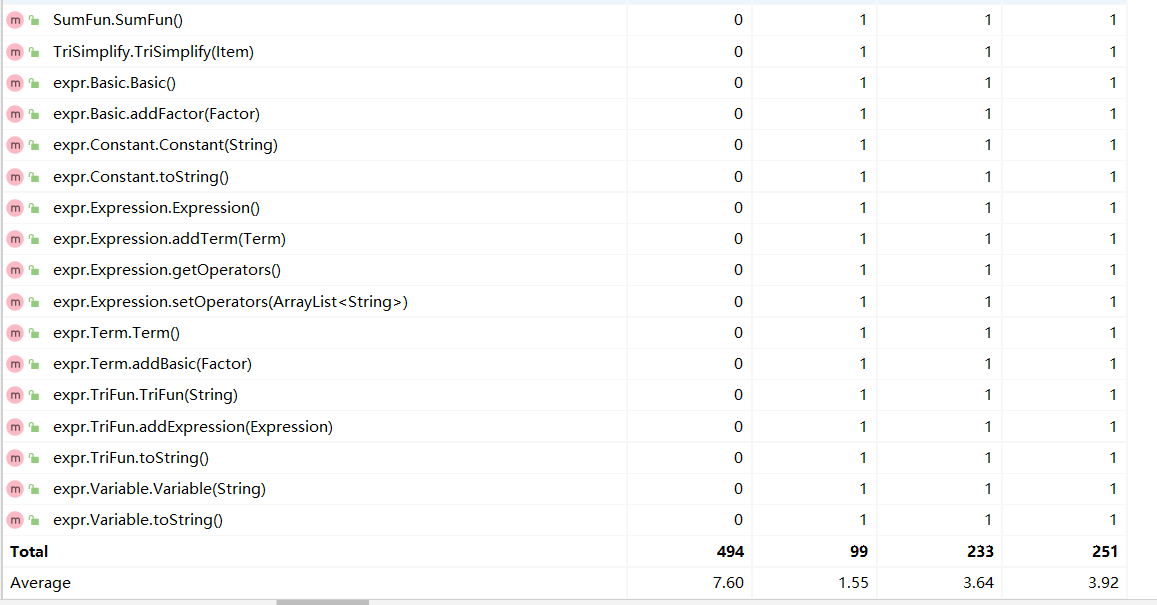• 类复杂度分析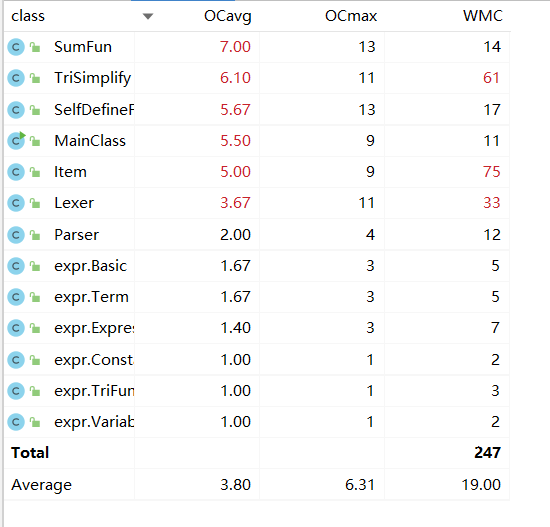1. <因子> 的长度为 1 , 则把 <因子>**2<因子>*<因子> 替换。 （Warning:若是三角函数中 "<因子>**2" 则不能应用此变换，原因为不符合本次作业中的形式化表述）
2. 把多项式的首个非负项放在表达式首位显示
3. 三角函数恒等变形（变形需要能使表达式长度缩减）
• 项*sin(<因子>)**2 + 项*cos(<因子>)**2  $$\rightarrow$$ 项
• sin(0) $$\rightarrow$$ 0cos(0) $$\rightarrow$$ 1
• sin(-<因子>) $$\rightarrow$$ -sin(<因子>) , cos(-<因子>) $$\rightarrow$$ cos(<因子>)
• a + b*sin/cos(<因子>)**2 $$\rightarrow$$ (a+b) - b*cos/sin(<因子>)**2
• 2 * sin(<常数因子>) * cos(<常数因子>) $$\rightarrow$$ sin(<常数因子> * 2)

## 第三次作业总结

### 需求变更

#### 数据限制变更:

• 三角因子嵌套：

• 支持因子嵌套在三角函数里面，并整体作为一个因子
• 函数嵌套

• 支持函数当做因子使用，并随意嵌套
• 数据量增加：

• 互测数据限制：Cost <= 1000 $$\rightarrow$$ Cost <= 100000, 允许出现求和函数

### 迭代开发

• #### 自定义函数类 SelfDefineFun• 新增该类的 check 方法用于检测并处理自定义函数调用中出现的递归调用问题

### 基于度量的程序结构分析

• 代码规模分析• 方法复杂度分析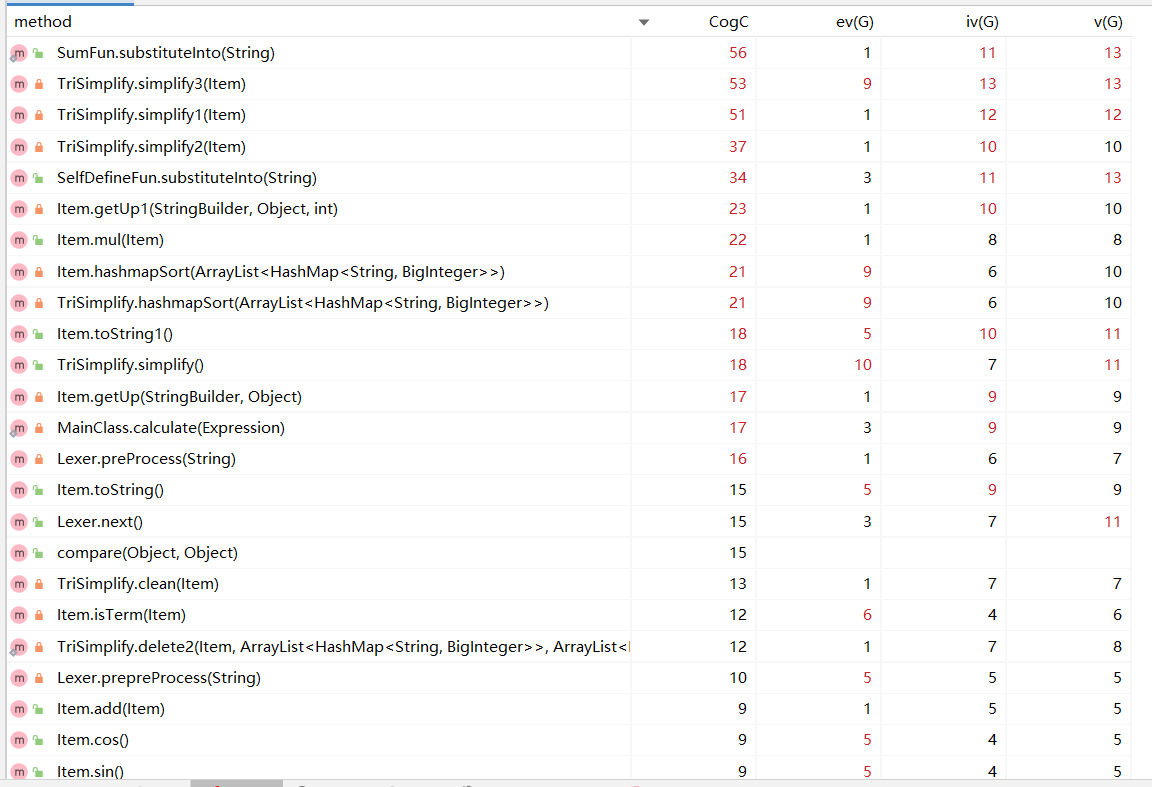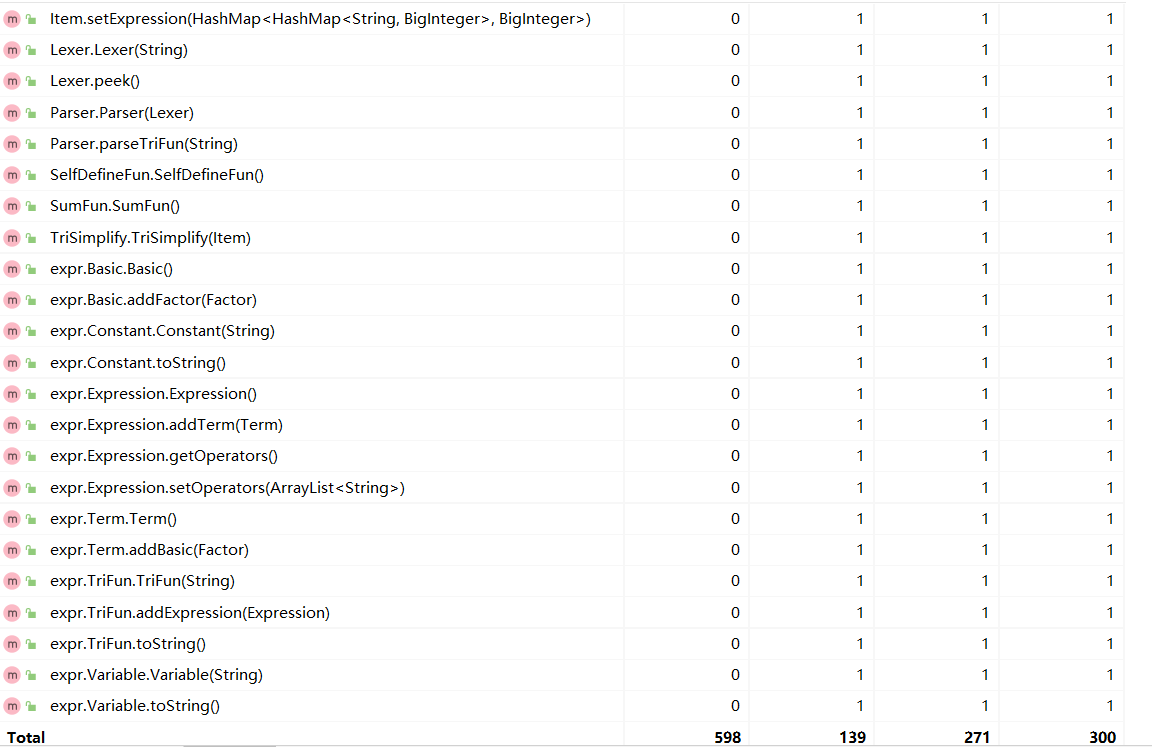• 类复杂度分析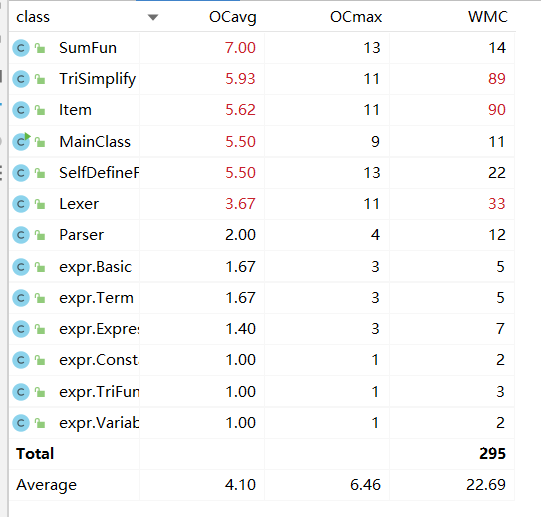1. <因子> 的长度为 1 , 则把 <因子>**2<因子>*<因子> 替换。
2. 把多项式的首个非负项放在表达式首位显示
3. 三角函数恒等变形（变形需要能使表达式长度缩减）
• 项*sin(<因子>)**2 + 项*cos(<因子>)**2  $$\rightarrow$$ 项
• sin(0) $$\rightarrow$$ 0cos(0) $$\rightarrow$$ 1
• sin(-<因子>) $$\rightarrow$$ -sin(<因子>) , cos(-<因子>) $$\rightarrow$$ cos(<因子>)
• a + b*sin/cos(<因子>)**2 $$\rightarrow$$ (a+b) - b*cos/sin(<因子>)**2
• 2 * sin(<常数因子>) * cos(<常数因子>) $$\rightarrow$$ sin(<常数因子> * 2)

## 分析自己程序的bug

• 本人三次作业强测及互测结果如图：

• 第一次作业：• 第二次作业：• 第三次作业：• 总共找出的 bug 数： 1

• 分别为：
1.

• 出错数据： sin((sin(x)*x))
• 错误答案： sin(sin(x)*x)
• 正确答案： sin((sin(x)*x))
• 出错原因： 计算时三角函数判定内部是否为表达式的方法 IsTerm() 判定条件错误

## 分析自己发现别人程序bug所采用的策略

• 根据形式化表述撰写 python 程序生成覆盖性测试数据对他人程序随机生成数据来进行黑盒测试

• 测试有效性： 较佳，互测基本能覆盖所有人被找出的bug

• 是否结合被测程序的代码设计结构来设计测试用例：

## 心得体会

###### author: 20373864 谭立德

posted on 2022-03-25 01:09  master-Tan  阅读(59)  评论(1编辑  收藏  举报